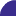SystemC AMS Building Block Library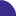In the course of the ANDRES project, a Library of configurable SystemC AMS modules was developed at the Vienna University of Technology, and is . The implemented building blocks focus on the field of communication and radio frequency systems, in particular on signal sources, modulation/demodulation blocks, filters, measurement and observation parts. They can be subdivided into three categories of blocks:

• Signal sources
• Basic blocks for signal processing
• Signal analysis units

The library also contains some example application. One example, an OFDM transceiver, is described here.

Examples (Full documentation available with package)

#### Gaussian distributed random Numbers

Functional description
This module generates Gaussian distributed random numbers. The parameters “_mean” and “_variance” are used to set the mean and variance of the random numbers. The data rate of the output port can be increased with the parameter “_rate”.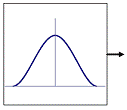Module definition:    noise_gauss(sc_module_name nm, double _variance, double _mean, int _rate);
Module Interface:     sca_tdf::sca_out<double> out;

Parameters:

 Name Type Default value Description nm sc_module_name - name of instant module _variance double 1.0 variance of random numbers _mean double 0.0 mean of random numbers _rate integer 1 data rate of output port

#### Low-noise amplifier (LNA)

Functional description
This module amplifies an input signal with a certain gain. User can set the gain with the parameter “_gain”. The non ideal effect “intercept modulation” can also be modelled with this module if necessary. With the parameter “_ideal” the user can switch between an ideal and a non-ideal LNA module. The IP3 point can be set with the parameter “_ip3”.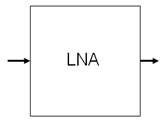Module definition:    lna(sc_module_name n, double _gain, double _ip3, bool _ideal);
Module interfaces:    sca_tdf::sca_in<double> in;
sca_tdf::sca_out<double> out

Parameters:

 Name Type Default value Description n sc_module_name - name of instant module _gain double - gain in dB _ip3 double - IP3 in dBm _ideal bool - true for simulation of ideal LNA  , otherwise false

#### OFDM modulator (OFDM transmitter)

Functional description
This module modulates a bit stream to an OFDM carrier signal, which is the sum of a number of orthogonal sub-carriers, with baseband data on each sub-carrier being independently modulated using quadrature amplitude modulation (QAM). The bit width of symbol can be set with the template parameter N.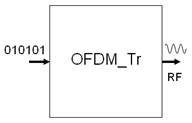Module definition:    template <int N>
ofdm_se(sc_module_name n,int qam_p_num,double mixer_fc,double bit_f,int dout_rate,double _amp)
Module interfaces:    sca_tdf::sca_in<bool> in;
sca_tdf::sca_out<double> out
Parameters:

 Name Type Default value Description nm sc_module_name - name of instant module qam_p_num integer - number of points in the constellation mixer_fc double - carrier frequency bit_f double - frequency of input bit stream data_rate integer - data rate of output port _ampl double 1.0 amplitude of carrier wave

## Example application: OFDM Transmitter system

The purpose of this chapter is to show the user how to use the modules from this building block library to model communication systems. We assume that the user who uses this library already has some basic knowledge of SystemC AMS.

Based on the previously described modules an OFDM transmitter system is built up (see Figure 11) in this Section.Top level block diagram of the example application

Figure 11 shows the structure of the transmitter system in our example. First, test data is generated by a test generator and passed to the transmitter. As the test generator the rand_bool module described in Section 2.3 is used and a serial of universal distributed random bits is generated. The transmitter takes these binary signals and modulates them to a high frequency OFDM signal (using Orthogonal Frequency Division Multiplex (OFDM) modulation). The ofdm_semodule described in Section 3.4.19 is used to model the transmitter. The generated signal is then passed via a channel (modelled using the air module described in 3.3.16) which attenuates its input signal and adds noise. After that the signal is taken by a receiver and translated back into a stream of binary bits.

In order to model communication systems using the modules in the library the user has to include the respective header data and set the right namespace first:

#include "tuv_ams_library/tuv_ams_library.h"
using namespace TUV_ams_lib::bb;

Then the expected modules from the library have to be instantiated in the following way:

rand_bool i_ i_stimuli.out(sig_stimuli); stimuli("stimuli",16);

where “rand_bool” is the module name, “i_stimuli” is the instance name of the module,  “stimuli” and “16” are values for respective parameters of the module.

Finally, SystemC AMS signals have to be declared to connect different modules:

sca_tdf::sca_signal sig_stimuli;
i_stimuli.out(sig_stimuli);

Here a signal called “sig_stimuli” is declared and connected to the “out” port of the module “i_stimuli”.

Apart from the above mentioned issues the user has to set the time resolution of the simulation using the predefined method “sc_set_time_resolution()”. It is also required to set the sampling rate on at least one port of the modelled system with the method “.set_T()”.

For the system described we need the following Section of codes:

```int sc_main(int argc, char* argv[])
{
sc_set_time_resolution(1, SC_PS);	// set the time resolution

sca_tdf::sca_signal<bool> sig_stimuli;    // declare SystemC AMS signals
sca_tdf::sca_signal<double> sig_out;
sca_tdf::sca_signal<double> noise_out;
...

rand_bool i_stimuli("stimuli",16);   // instantiate test generator
ofdm_se<8> i_tran("transmitter",constl_dim,
freq_carrier,freq_bit,data_rate,ampl_se); // instantiate transmitter
air i_air("air",attent,"gauss_white",n_va,n_mean,data_rate);
// instantiate channel
drain drn("drn");                    // instantiate drain. This is only a
// module used to consume tokens as we
// can not let a SystemC AMS scheduling
// loop open.

i_stimuli.out.set_timestep(1/freq_bit,SC_SEC); //Time step has to be set to one of
the ports in the system

i_stimuli.out(sig_stimuli);          // connect different modules
i_tran.in(sig_stimuli);
i_tran.out(sig_out);
...

// Tracing:
sca_util::sca_trace_file wave = sca_util::sca_create_vcd_trace_file ("wave");
sca_util::sca_trace (wave, sig_stimuli ,"stimuli");
...

sc_start(0.05,SC_MS);                // Simulation time: 0.05ms
return 0;
}```

(This is not the complete source code)

The transmitter and receiver modules consist again of sub modules, which are contained in the library. Figure 12 and Figure 13 present the internal structure of the transmitter module and receiver module, respectively.

The transmitter takes a serial stream of binary digits. By inverse multiplexing, these are first demultiplexed into N parallel streams, and each one mapped to a (possibly complex) symbol stream using QAM modulation.

An inverse FFT is computed on each set of symbols, giving a set of complex time-domain samples. These samples are then quadrature-mixed to passband in the standard way.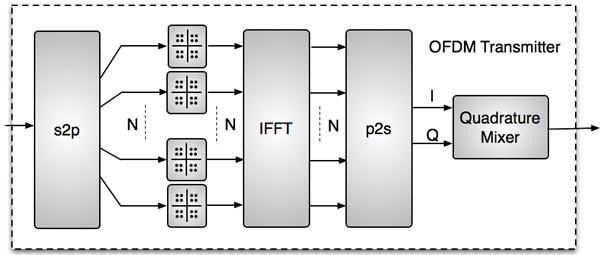Block diagram of OFDM transmitter

The receiver picks up the signal from antenna, which is then quadrature-mixed down to baseband using cosine and sine waves at the carrier frequency. This also creates signals centered on 2fc, so low-pass filters are used to reject these. The baseband signals are then sampled and a forward FFT is used to convert back to the frequency domain. This returns N parallel streams, each of which is converted to a binary stream using an appropriate symbol detector. These streams are then re-combined into a serial stream, which is an estimate of the original binary stream at the transmitter.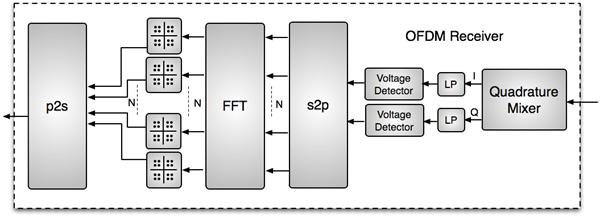The next code Section shows how to use building block modules to build the OFDM transmitter module:

```template <int N>
SC_MODULE(ofdm_se){
public:
//Ports:
sca_tdf::sca_in<bool> in;            // declare port
sca_tdf::sca_out<double> out;
//signals
sca_tdf::sca_signal<bool> sig_pa[N];  	// declare signals to
sca_tdf::sca_signal<double> sig_real[N];  // connect sub modules
...
private:
//module instantiation
s2p<bool,N>* s2p_sub;
qam_map* qam_mapper_sub[N];
fft_ifft<N>* ifft_sub;
p2s<double,N>* p2s_r_sub;
p2s<double,N>* p2s_i_sub;
q_mixer_tr* q_mixer_tr_sub;
public:
//Constructor
ofdm_se(sc_module_name n,int qam_p_num,double mixer_fc,double bit_f,int
dout_rate,double _amp=1)
{
int mixer_rate=(int)floor(dout_rate*log2(qam_p_num)*mixer_fc/bit_f);
// instantiating modules and connecting them using signals
s2p_sub = new s2p<bool,N>("s2p_sub",1);  // serial to parallel module
s2p_sub->in(in);
for(int i=0;i<N;i++)
s2p_sub->out[i](sig_pa[i]);

...

ifft_sub = new fft_ifft<N>("ifft_sub","IFFT"); //IFFT module
for(int i=0;i<N;i++)
{
ifft_sub->in_real[i](sig_real[i]);
ifft_sub->in_imag[i](sig_imag[i]);
ifft_sub->out_real[i](sig_out_real[i]);
ifft_sub->out_imag[i](sig_out_imag[i]);
}

...

p2s_r_sub = new p2s<double,N>("p2s_r_sub",1); //quadrature mixer module
q_mixer_tr_sub = new q_mixer_tr("q_mixer_tr_sub",mixer_fc,_amp,0.,0.,mixer_rate,false);
q_mixer_tr_sub->i_in(sig_out_i);
q_mixer_tr_sub->q_in(sig_out_q);
q_mixer_tr_sub->out(out); /***/

}
};

```

As we can see from the source code the modelling of an OFDM transmitter is quite easy when using the existing modules in the library.

Simulation example:
In this Section several simulation results are presented with different settings of the following parameters:

• constl_dim, defines the number of points in the QAM constellation
• ampl_se , defines the amplitude of the carrier for the transmitter
• ampl_re, defines the amplitude of the carrier for the receiver
• n_amp, maximal value of noise in channel
• atten, attenuation of channel

1. without noise and attenuation in channel:

constl_dim = 16,ampl_se=1.0,ampl_re=1.0,n_amp=0.0,atten=1.0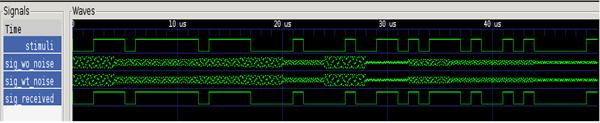1. with gaussian noise ,without attenuation in channel:

constl_dim = 16,ampl_se=1.0,ampl_re=1.0,n_amp=90.0,atten=1.0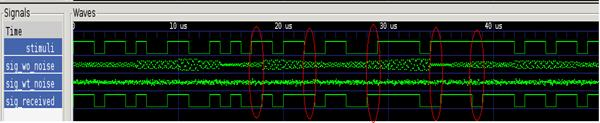As we can see that because of the noise in the channel we got 5 error bits.

1. with gaussian noise and 50% attenuation in channel:

constl_dim = 16,ampl_se=1.0,ampl_re=1.0,n_amp=90.0,atten=0.5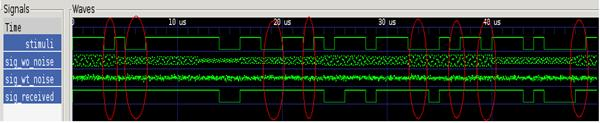Having both noise and attenuation in the channel the number of error bits became larger.

1. with gaussian noise and 50% attenuation, but with higher transmission power

constl_dim = 16,ampl_se=20,ampl_re=0.1,n_amp=90.0,atten=1.0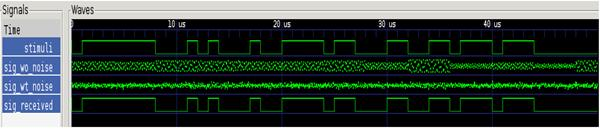As presented in this figure we can reproduce the correct signals with higher transmission power. This is due to the parameter “ampl_se” set to 20, which means the signal from the transmitter is 20 times as strong as by previous simulations.

1. with gaussian noise and 50% attenuation, but slowing down transmission speed

constl_dim = 4,ampl_se=1.0,ampl_re=1.0,n_amp=0.0,atten=1.0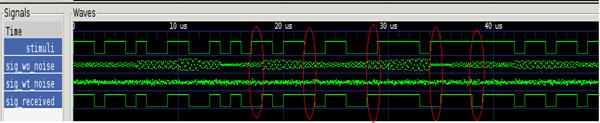Or it is also possible to slow down the transmission speed to improve the BER. This is due  to the parameter “constl_dim” set to 4, which means now only 2 bits (which is 4 bits when setting “constl_dim” to 16) were encoded at one time.